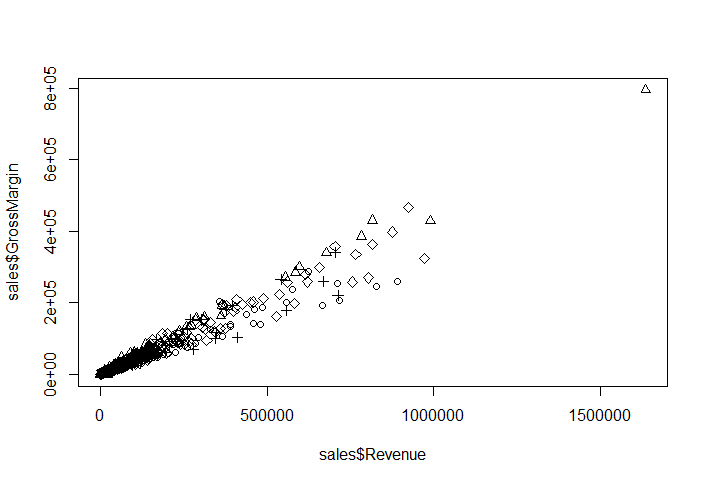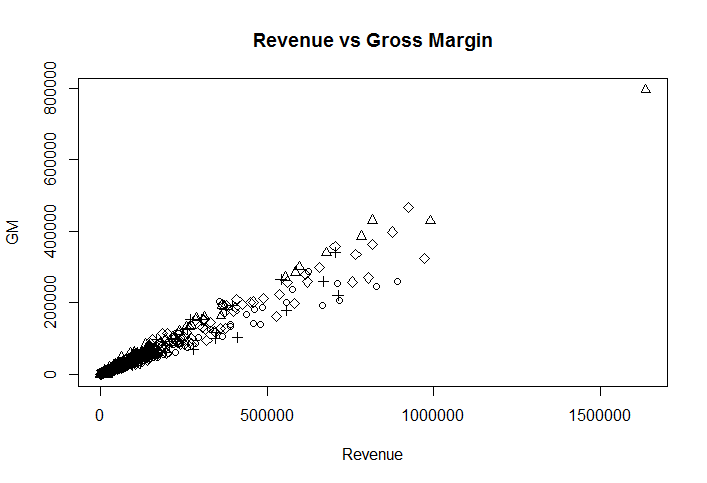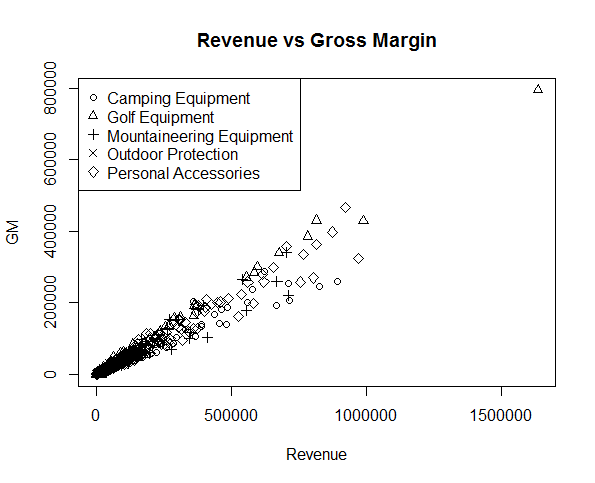# Create a Scatter Plot in R with Multiple Groups

Let's say you have Sales Orders data for a sports equipment manufacturer and you want to plot the Revenue and Gross Margins on a scatter plot. However, you also have a ProductLine column that contains information about the product category and you want to distinguish the x,y points by the ProductLine.

We can do so using the pch argument of the plot function.

> plot(x, y, pch=as.integer(f))


By specifying this option, the plot will use a different plotting symbol for each point based on its group (f).

We have created a sample dataset for this lesson which contains Sales, Gross Margin, ProductLine and some more factor columns. You can download this dataset from the Lesson Resources section. As always, we will first load the dataset into an R dataframe.

> setwd("C:/r-programming/data")
> getwd()


Before plotting the graph, it's a good idea to learn more about the data by using the summary() and head() functions.

We are interested in three columns from this dataset:

• Revenue: The total revenue from each order. We will plot this on x-axis.
• Gross Margin: The gross margin from each order. We will plot this on y-axis.
• ProductLine: The product category. We will group the data by ProductLine.

We can now draw the scatter plot using the following command:

plot(sales$Revenue,sales$GrossMargin,pch=as.integer(sales$ProductLine))  The result is displayed below. You can clearly see the points with different symbols according to their group.Notice that R has converted the y-axis scale values to scientific notation. We can correct this by changing the option scipen to a higher value. This controls which numbers are printed in scientific notation. > options("scipen" = 10) > options()$scipen
 10


If you plot the chart again, the numbers would display correctly.Now that we have different symbols being used for different groups, we can make the graph even more convenient by adding a legend to it. We can do so by calling the legend function after the plot function.

legend(x, y=NULL, legend, …)


x, y are the coordinates for the legend box. There are two ways to specify x: 1) Specify the position by using "topleft", "topright", etc. 2) Use an x-coordinate for the top-left corner of the legend. If you choose option 1 for specifying x, then y can be skipped. Alternatively you need to specify the y-coordinate for the top-left corner of the legend.

The third argument "legend" is a vector of the character strings to appear in the legend.

You also need to specify a fourth argument that varies depending on what you're labeling. You can create legends for points, lines, and colors. In our case, we are creating legend for points, so we will provide the forth argument pch which is also a vector indicating that we are labeling the points by their type.

> legend("topleft", c("Camping Equipment","Golf Equipment","Mountaineering Equipment", "Outdoor Protection", "Personal Accessories"), pch=1:5)


The graph will now look as follows:The legend function can also create legends for colors, fills, and line widths.The legend() function takes many arguments and you can learn more about it using help by typing ?legend.

### Exercise

• Download and load the Sales_Products dataset in your R environment
• Use the summary() function to explore the data
• Create a scatter plot for Sales and Gross Margin and group the points by OrderMethod
• Add a legend to the scatter plot
• Add different colors to the points based on their group. (Hint: Use the col argument in the plot() function

#### Lesson Resources

Get our Data Science for Finance Bundle for just $29$51.